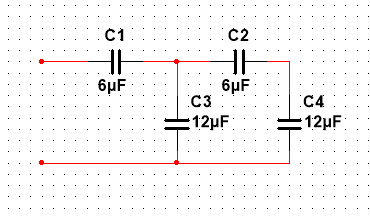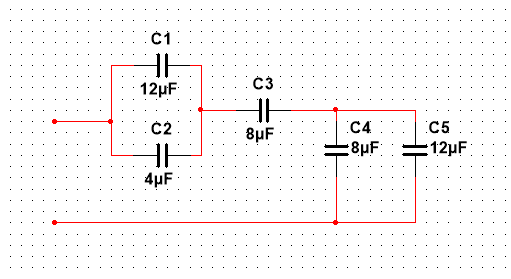# MODULE 7: CAPACITORS AND INDUCTORS M7A1 Solved

20.00 \$ 10.00 \$

Category:
Click Category Button to View Your Next Assignment | Homework

You'll get a: . ` pdf` file solution, download link after Payment

## Description

5/5 - (1 vote)

Problem 1. Calculate the value of a capacitor with a charge of 3600 µC when the voltage across its plates is 48 V.

Problem 2. Calculate the electric field between the plates of a 4.7 µF capacitor that are separated 4 mm, with 160 µC of charge.

Problem 3. Calculate the capacitance seen from the open terminals in the circuit below:Problem 4. Calculate the capacitance seen from the open terminals in the circuit below:Problem 5. Consider a 1000 µF capacitor charged to 100 V.

1. a) Calculate the energy stored in the capacitor.
2. b) Find the charge in the capacitor.
3. c) If the capacitor is fully discharged in 0.5 ms, what is the average current flowing through the capacitor?